# How to Add or Subtract Times to Get Hours/Minutes/ Seconds in Excel?

Sometimes, when we add or subtract different times, we want to get the result only in hours, minutes, or seconds. When we add or subtract time from time, we typically get the following result: hour: minutes: seconds. But we can make some changes in Excel in order to get the result only in seconds, minutes, or hours. This tutorial helps you understand how we can make it happen.

## Adding or Subtracting Times to Get Hours/Minutes/Seconds In Excel

Here we will first add the values and use the format to complete the task. Let's look at a simple method for adding or subtracting time. This process includes two steps: first, we will use the sum function to add time, and then format the cells to get the result.

Step 1

Let us consider the Excel sheet, which has data that is similar to the below screenshot.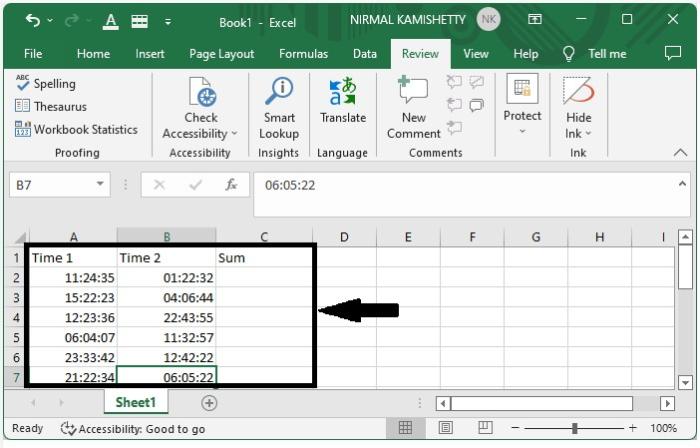To add the two times, we can use the SUM function. Enter =SUM(A2+B2) in the formula box of cell C2 and press Enter to get the first result.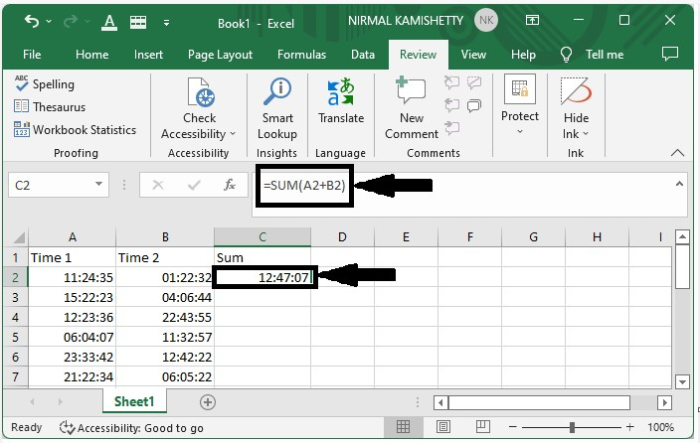Step 2

We can get all the other results just by dragging down from the first result until all the results are filled, and it will look like the below image.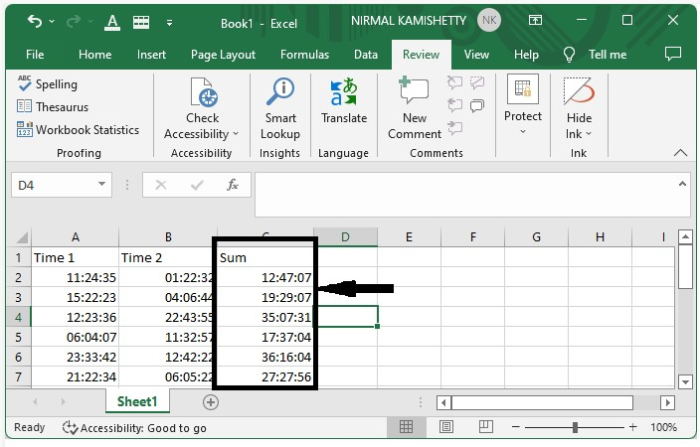If the time is longer than 24 hours, select the sum and enter the format [h]:mm:ss;

Step 3

To represent the data in hours, select the data, right-click on it, and then select format cells. A new pop-up window will open, and we can enter the formula [hh] in the custom menu, then click OK, as shown in the image below.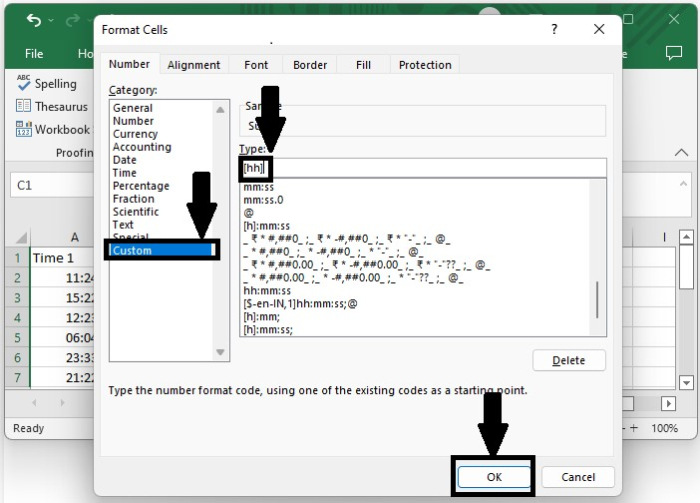And the final result will look very similar to the image below.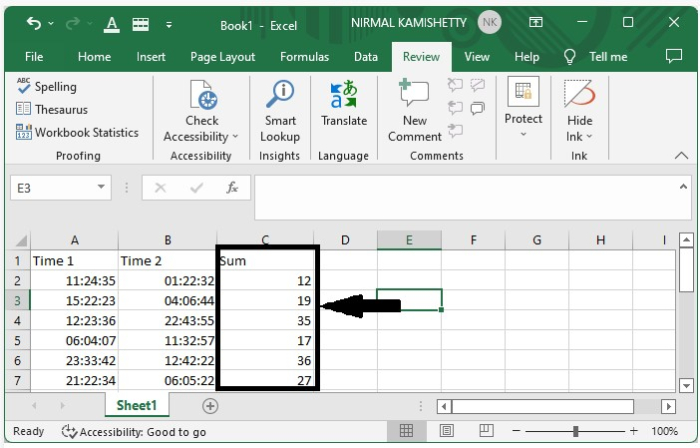To get minutes, type [mm]; for seconds, type [ss] in the type box.

## Conclusion

In this tutorial, we used a simple example to demonstrate how you can add or subtract times to get hours, minutes, and seconds in Excel to highlight a particular set of data.

Updated on: 09-Jan-2023

513 Views